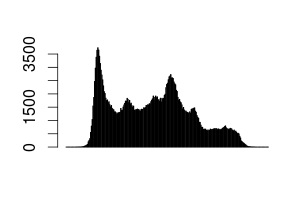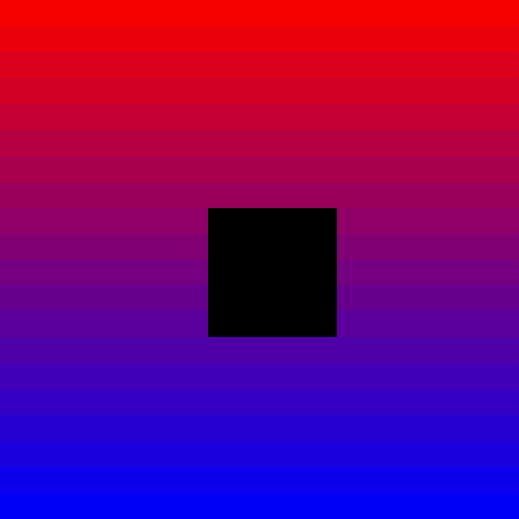# 7. RRasdaman Guide¶

RRasdaman is an R package providing database interface for rasdaman.

## 7.2. Connect to rasdaman¶

The first thing to do is to establish a connection to the rasdaman database from R:

library(RRasdaman)
conn <- dbConnect(Rasdaman())


Connection is the object which allows to send queries to database and to manage transactions. One can also specify host, port, username and password which should be used for the connection. Additionally, connections could be read-only and writable. The full signature of the dbConnect method and its default arguments are the following:

dbConnect(drv, host = "localhost", port = 7001,
dbName = "RASBASE", user = "rasguest",


Do not forget to close your connection with dbDisconnect(conn) after the work is done! You always can list all open connections with . Here is an example on how to create several connections and close them in one command:

> conn1 <- dbConnect(Rasdaman())
> conn2 <- dbConnect(Rasdaman())
> dbListConnections(Rasdaman())
[]
An object of class "RasdamanConnection"
Slot "jObj":
 "Java-Object{RasConnection{ host=localhost, port=7001, db=RASBASE, user=rasguest, mode=READ_ONLY, alive=true }}"

[]
An object of class "RasdamanConnection"
Slot "jObj":
 "Java-Object{RasConnection{ host=localhost, port=7001, db=RASBASE, user=rasguest, mode=READ_ONLY, alive=true }}"

> lapply(dbListConnections(Rasdaman()), dbDisconnect)
> dbListConnections(Rasdaman())
list()


## 7.3. Handles¶

After the connection is established, we can send our first query and read some data from rasdaman. Let’s compute the average temperature over the whole observation period from the “climate_temperature” dataset:

handles <- dbGetQuery(conn, "select avg_cells(x) from climate_temperature as x")
length(handles)
#  1
simplify(handles[])
#  272.1054


First, we send a query to rasdaman with dbGetQuery in rasql language. The result is a list of handles for each result of query execution over arrays in the collection. A handle represents the query execution result which is not yet converted into R data representation. In our case there is only one array in the collection “climate_temperature”, so we have only one result, so we have only one handle.

Then we convert the returned handle into an R object with the simplify method and can see the scalar result.

## 7.4. Arrays representation¶

Now let’s look what happens if we retrieve some array, not the single scalar value.

> handles <- dbGetQuery(conn, "select (RGBPixel) x[0:1,0:1] from rgb as x")
> obj <- simplify(handles[])
> obj
An object of class "RasdamanArray"
Slot "array":
$red [,1] [,2] [1,] 169 168 [2,] 169 168$green
[,1] [,2]
[1,]  210  209
[2,]  210  209
$blue [,1] [,2] [1,] 212 211 [2,] 216 213 Slot "origin":  0 0  As we can see, the result is an object of S4 class RasdamanArray. It has two slots: array and origin, which could be accessed as obj@array and obj@origin. The origin of an array is the coordinates of its lowermost leftmost cell. The obj@array slot is a list of N-D matrices, each matrix represents one of N attribute. For example, one can use both obj@array$green and obj@array[] to access the green channel values, as green is the second component of the RGB structure.

## 7.5. Displaying data¶

Rasdaman has a flexible query language, so in many cases it is possible to compute sophisticated expressions on the server side rather than doing them in R. For example, one can fetch the full dataset into R session and build the histogram over its values, but it is also possible compute histogram with rasql. The second approach requires less data to be transmitted between the rasdaman server and the client application, and the client needs less memory to store it. In the following example we build the histogram of pixel intensities:

handles <- dbGetQuery(conn, "select marray n in [0:255] values count_cells(c = n) from lena as c")
data <- simplify(handles[])
values <- data@array[]
barplot(values)## 7.6. Writing data¶

If one wants to write some data to rasdaman, a connections with write permissions needs to be created:

conn <- dbConnect(Rasdaman(), user="rasadmin", password="rasadmin", mode=CONN_READ_WRITE)


We can create a collection with regular rasql syntax. Let’s create a collection images of 2-D RGB data:

dbGetQuery(conn, "create collection images RGBSet")


Now we need data to be inserted into the newly created collection. Let’s generate some image of size 20x20 with smooth gradient from red to blue. The origin point (i.e. leftmost lowermost coordinate of the array) will be [0,0].

n <- 20
m <- 20
red <- array(seq(247,0, length.out=n), c(n, m))
green <- array(0, c(n, m))
blue <- array(seq(0, 247, length.out=n), c(n, m))
origin <- as.integer(c(0,0))
arr <- RasdamanArray(list(red=red, green=green, blue=blue), origin)


This generated array can be inserted into the collection with method dbInsertCollection:

dbInsertCollection(conn, name="images", value=arr, typename="RGBImage")
#  420865


We need to specify collection name, array to be inserted and the type of the array. The method returns one number – the object identifier of the newly inserted array.

Now let’s update the inserted data. We will set to zero all values in the middle of the newly inserted array. For this we need to create an array with new values.

zeroes <- array(0, c(5,5))
origin = as.integer(c(8,8))
updArray <- RasdamanArray(list(red=zeroes, green=zeroes, blue=zeroes), origin)
dbUpdateCollection(conn, name="images", value=updArray, typename="RGBImage")


After the collection is updated, we can read the values, display the result and close the connection:

handles <- dbReadCollection(conn, "images")
result <- simplify(handles[])

library(grid)
picture <- rgb(result@array[], result@array[], result@array[], max=255)
dim(picture) <- dim(result)
grid.raster(picture, interpolate=F)
dbDisconnect(conn)The list of allowed type names could be obtained with a rasql query, see Type Definition Using rasql.

## 7.7. Transaction management¶

The methods dbCommit and dbRollback could be used for transaction management. There is no need to explicitly specify beginning of the transaction. The method dbDisconnect commits the transaction before closing the connection.

You can type ?RRasdaman::RRasdaman and help.search("RRasdaman") at any time from R prompt to see more package documentation.